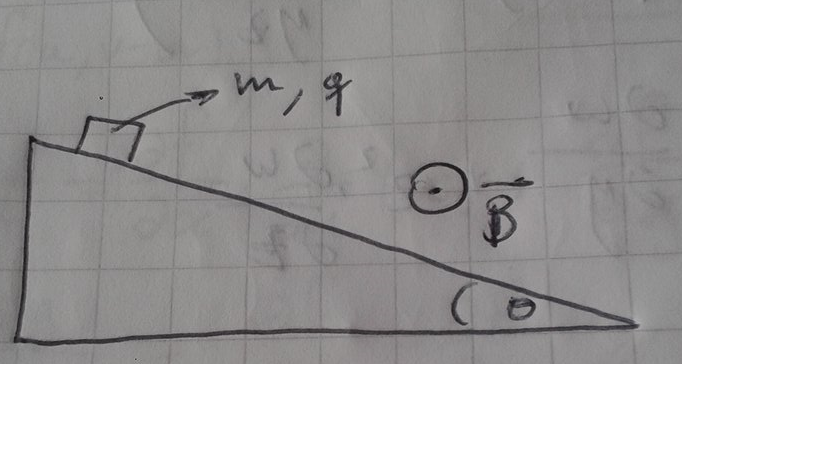# The Field Keeps Me DownAn object with mass $m$ and charge $q$ is dropped on an infinite inclined plane which forms an angle $\theta$ with the horizontal, in the presence of a constant magnetic field $B_{0}$ which goes out the page. If the coefficient of friction between the plane and the object is $\mu$, the maximum magnetic force can be written as $\dfrac{mg}{\mu}(a-\mu b)$, find the value of $a^2+b^2$

×